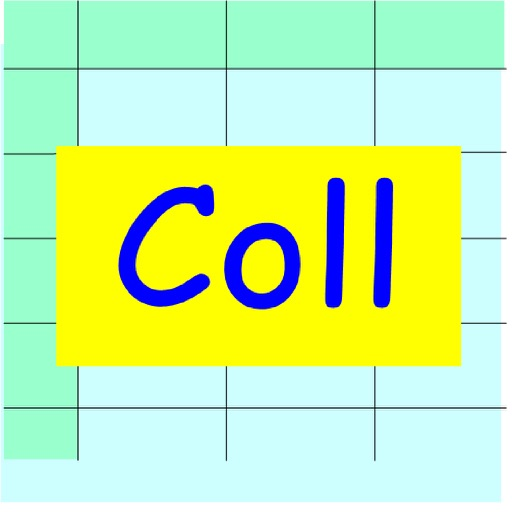Collision Calculator# Collision Calculator

## Mineo Yamauchi

1.02 for iPhone
\$0.99
0

0 Ratings

Release Date

2016-12-12

Size

0.4 MB

### Screenshots for iPhone

iPhone
Collision Calculator Description
Collision Calculator is a software that solves one dimensional collision problem of two objects. It performs the following calculations. When two initial velocities are known, get two final velocities after collision. When two final velocities are known, get two initial velocities before collision. When two initial velocities and one final velocity are known, get another final velocity and coefficient of restitution COR).
It's possible to change one of input data one after another. Thus it's possible to get output data which correspond to changed input data.

（Example)
A first ball of mass 1 Kg is moving with the velocity 1 m/s, and second ball of mass 2 Kg is stationary. Calculate the velocities of both ball after collision.
When the first ball velocity is changed to 0.9, 0.8, and 0.7 m/s, calculate the velocities of both ball after collision.
Assume that coefficient of restitution is 0.5.

Enter as follows,
e = 0.5
M1 = 1, V1 = 1
M2 = 2. V2 = 0

Touch out P button that corresponds to the data which is planning to be changed.
We are planning to change the velocity of the first ball.
Touch out V1_P button.

We obtain 0.0 m/s and 0.5 m/s as the final velocities.

Delta data is attached to the V1 data as an increment.
Enter as follows.
Delta = 0.1

Touch out - button of the V1 data. V1 data is decremented.

We obtain 0.0 m/s and 0.45 m/s as the final velocities that correspond to the first ball velocity 0.9 m/s.
Next touch out - button. We obtain the next output data.
Collision Calculator 1.02 Update
2016-12-12 Version History
Updated for iOS10 and the new SDK of Xcode8.
Added example-3 Super elastic collision
Added example-4 Required bat speed change for COR change.
More
Price:
\$0.99
Version:
1.02
Size:
0.4 MB
Genre:
Education Games
Release Date:
2016-12-12
Developer:
Mineo Yamauchi
Language:
English
More
You May Also Like
Developer Apps